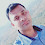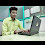# History & scope of Python

57

### Python Script performance is best as compare to other languages means its performance is much better as compared to C, CPP, Java, PHP, and Another Programming language. Python script only focuses on a set of code .it not use code pattern and code structure.

.....................................................................................................................................................

### How to run the Python Script:-

1 Online Mode:-
We can run a python script without using any software because Python Software tools are online available.
www.jupyter.org/try

........................................................................................................

Offline Mode:-
double click and execute them.
After Successfully installation Python Provide Default IDLE Tools
...............................................................................................................................................................

Python Program Execution:-
1 Python Source Code ------> Run  -----> PYTHON INTERPRETER MACHINE ----> Machine Code----> Output
.....................................................................................................................................

Program to calculate simple interest?
p,r,t=12000,2.2,3.5
si=(p*r*t)/100
print("result is p={0} , r={1} , t={2} ,si={3} ".format(p,r,t,si))
#print("result is %.2f" % si)
Input /Output function for Python Console Application:-

Output Function
print():-   it is used to print a single line statement and multiple line statements both.
print("hello")    #single statement
print("hello","world")  #Multiple Statement
print("hello" + "world")  #Conacatenation
print(" %f " %  1.2345)
input():-  it is used to take input from the user's.
var=input("message")
input return data in String format
a= int(input("message"))
a=int(input("enter first number"))
b=int(input("enter second number"))
c=a+b
print(c)
#ASSIGNMENT:-
WAP to Calculate the Area of Triangle and Rectangle?
WAP to Reverse Five Digit Number?
WAP to Calculate Addition of Complex Number?
Solution:-
num=78125
print("Actual number is",num)
a=num%10  #5
num=num//10 #1234
b=num%10    #4
num=num//10 #123
c=num%10    #3
num=num//10 #12
d=num%10    #2
e=num//10   #1
num1= a*10000+b*1000+c*100+d*10+e*1
print("Reverse of number is",num1)
Addition of complex numbers without using complex()?
r1 = 2
i1 = 3
r2=5
i2=7
r = r1+r2
i = i1+i2
print("{0}+{1}j".format(r1,i1))
print("{0}+{1}j".format(r2,i2))
print("{0}+{1}j".format(r,i))
Addition of a complex number with a complex number:-
num1 = 2+3j
num2 = 4+5j
num = num1+num2
print(num1)
print(num2)
print(num)
Tags

1.area of traingle
b,h=10,20
Area=(b*h)//2
print(Area)

2.area of rectangle
w,l=20,30
Area=(20*30)
print(Area)

3.adding complex number using complex number
A=2+3J
B=3+4J
C=A+B
print(A)
print(B)

4.print("Knowing Base and Height")
h = int(input("Enter height of Triangle :\t"))
b = int(input("Enter length of the Base :\t"))
c = b*h/2
print("\n Area of a Triangle",c)

5.#Area of a Rectangle
print(" If length and Width are given")
b = int(input("Enter Width of Rectangle :\t"))
l = int(input("Enter length of Rectangle :\t"))
c = l*b
print("\n Area of a Rectangle",c)

6.#Code for Reversing Five Digit Number
f = str(input("Enter Five Digit No. :\t"))

l=f[::-1]

7.i = int(input("enter a number :"))
rev = 0
while (i>0):
rev=(rev*10)+i%10
i=i//10
print("revers=",rev)

8.# Three sides of the triangle is a, b and c:
a = float(input('Enter first side: '))
b = float(input('Enter second side: '))
c = float(input('Enter third side: '))

# calculate the semi-perimeter
s = (a + b + c) / 2

# calculate the area
area = (s*(s-a)*(s-b)*(s-c)) ** 0.5
print('The area of the triangle is %0.2f' %area)

9.first = complex(input('enter first complex number: '))
second = complex(input('enter second complex number: '))

10.Area of traingle
B=10
H=30
Area =(B*H) /2
Print ("Area")

11.Area of rectangle
W, L=20, 25
Area=(W*L)
Print("Area")

12.***Area of Triangle***
base=int(input("enter the base of traingle"))
height=int(input("enter the height of triangle"))
area=((base*height)*1/2)
print("area= %d"% area)

13.***Area of Rectangle***
width=int(input("enter the width of ractangle = "))
height=int(input("enter the height of ractangle = "))
area=width*height
print("area= %d"% area)

14.a=2+3j
b=3+2j
c=a+b
print(c)

15.*** 5 digit reverse***
a=int(input("enter the 5 digit no = "))
i=a%10
a=int(a/10)
j=a%10
a=int(a/10)
k=a%10
a=int(a/10)
l=a%10
a=int(a/10)
print(str(i)+str(j)+str(k)+str(l)+str(a))

16.#MOhit Chouhan
Question 1.
Area Of Triangle
B=4
H=5
area= (1/2*(B*H))
print("The Area of Triangle is " , area)

Area of rectangle
W=20
L=30
Area=(W*L)
print(Area)

Ques.2
a=12345
i=a%10
print(i) #5
a=int(a/10)
print(a) #1234
j=a%10
print(j)#4
a=int(a/10)
print(a) #123
k=a%10
print(k)#3
a=int(a/10)
print(a)#12
l=a%10
print(l)#2
a=int(a/10)
print(a) #1
print(str(i)+str(j)+str(k)+str(l)+str(a))

a=5+2j
b=2+5j
c=a+b
print(c)

17.#Mohit Singh Chouhan
a= 12345
b=a%10 #5
v=a//10 #1234
c=v%10 #4
w=v//10 #123
d=w%10 #3
x=w//10 #12
e=x%10 #2
y=x//10 #1

print(str(b)+str(c)+str(d)+str(e)+str(y))

18.Area of Triangle
j= 6
>>> k= 8
>>> area= (1/2*(j*k))

area of rectangle
l= 10
>>> b= 5.5
>>> a= l*b
>>> print(a)
>>> print(area)

19.reverse five digit of 12345

a=12345
num=0
num=(a%10)*10
a=a//10

num=(num+(a%10))*10

a=a//10
num=(num+(a%10))*10
a=a//10
num=(num+(a%10))*10
a=a//10
num=(num+(a%10))

print(num)

20.>>> a= 4+5j
>>> b= 5+6j
>>> c= a+b
>>> print(c)
(9+11j)

21.#WAP to Reverse Five Digit Number?
num=10021
a=num%10 #1
num=num//10 #
b=num%10 #2
num=num//10 #
c=num%10 #0
num=num//10 #
d=num%10 #0
num=num//10 #
e=num%10 #1
num1=(a*10000+b*1000+c*100+d*10+e*1) # 10000 is place value
print(num1)

22.#1 wap to addition complex number
com=4+5j
com1=6+15j
com2=com+com1
print(com2)

# 2 wap to find the reminder
# %( reminder)
a=22
b= a%3
print(b)

23.#WAP to calculate the area of a triangle, circle, and rectangle ?
h=15
b=20
l=30
r=10
pi=3.14
p=int(input("press 1 for triangle and press 2 for circle and press 3 for rectangle :- "))
triangle=(h*b)/2
circle=pi*r*r
rectangle=l*b
if p==1:
print("Area of triangle ",triangle)

if p==2:
print("Area of circle ",circle)

if p==3:
print("Area of rectangle ",rectangle)

24.#SHREYA SINGH
#WAP to Reverse Five Digit Number?

num=12345
print("Given Number is:",num)
a=num%10 #5
num=num//10 #1234
b=num%10 #4
num=num//10 #123
c=num%10 #3
num=num//10 #12
d=num%10 #2
e=num//10 #1
rev=a*10000+b*1000+c*100+d*10+e*1
print("Reverse of the Number is:",rev)

25.Addition of complex numbers without using complex()?
a=int(input("enter real number:-"))
bj=int(input("enter imaginary number:-"))
c=int(input("enter real number:-"))
dj=int(input("enter imaginary number:-"))
r=a+c
r1=bj+dj
print("{0}+{1}j".format(a,bj))
print("{0}+{1}j".format(c,dj))
print("{0}+{1}j".format(r,r1))

26.h,b=int(input("enter heigth of triangle:-")),int(input("enter base of triangle:-"))
area=(h*b)/2
print("area of triangle is:-",area)
l,w=int(input("enter length of rectangle:-")),int(input("enter width of ractangle:-"))
area1=l*w
print("area of ractangle is:-",area1)

27.#reverse number using while loop
num=int(input("enter any number:="))
print("reverse num is:-")
while num>0:
print(num%10)
num=num//10

28.# reverse 5 digits number without while loop
mun=int(input("enter any number:-"))
a=mun%10
mun=mun//10
b=mun%10
mun=mun//10
c=mun%10
mun=mun//10
d=mun%10
mun=mun//10
e=mun%10
mun=mun//10
print(a,b,c,d,e)

29.#Area of Tringle
b=10
h=20
Area=(b*h)/
/2
print(Area)

30.#Adding complex number using complex number

A=2+4J
B=4+5J
c=A+B
print(A)
print(B)
print(c)

31.sonam singh

a=10
>>> b=20
>>> area=(a*b)//2
>>> print(area)

32.sonam singh
a=10
>>> b=20
>>> area=(a*b)//2
>>> print(area)

33.SONAM SINGH
>>> a=5
>>> print(a*a)
25
>>> print(a*a*a)
125
>>>

34.Area of Traingle

B=int (input("enter the first no ;"))
H=int (input("enter the second no ;"))
Area=(B*H)/2
print(Area)

35.SONAM SINGH
a=145
>>> num1=a%10
>>> a=a//10
>>> num1=a%10
>>> format(num1)
'4'
>>> b=154
>>> num2=a%10
>>> b=b//10
>>> num2=b%10
>>> format(num2)
'5'
>>> c=451
>>> num3=c%10
>>> c=c//10
>>> num3=c%10
>>> format(num3)
'5'
>>>

36.SONAM SINGH
base=int(input("enter the base value"))
height=int(input("enter the height value"))
area=(base*height)//2
print(area)

37.#Abhishek parihar

rn1=3
in1=4j
rn2=5
in2=6j
realnumber=(rn1+rn2)
imaginerynumber=(in1+in2)
print(realnumber+imaginerynumber)

38.#WAP to Calculate the Area of Triangle and Rectangle?
#area of triangle = 1/2*b*h
#area of circle= 3.14*r**2
b=float(input("enter the base"))
h=float(input("enter the hight"))

aot=(1/2)*b*h

aoc=3.14*r**2
print("the area of triangle and area of circle is", aot , aoc)

39.#WAP to Reverse Five Digit Number?
num=int(input("enter five digit no."))
a=num%10
num=num//10
b=num%10
num=num//10
c=num%10
num=num//10
d=num%10
e=num//10
rev=a*10000+b*1000+c*100+d*10+e*1
print("the reverse value is",rev)

40.#WAP to Calculate Addition of Complex Number?
#6+9j,10+4j
A=6+9j
B=10+4j
C=A+B
print(A)
print(B)
print("the addition of complex no. is",C)

41.r1=4
i1=3
r2=3
i2=5
r=r1+r2
i=i1+i2
print("{0}+(1)j".format(r1,i1))
print("{0}+{1}j".format(r2,i2))
print("{0}+{1}j".format(r,i))

42.#WAP to Calculate the Area of Triangle and Rectangle?
#area of triangle = 1/2*b*h
#area of rectangle= wl
b=float(input("enter the base"))
h=float(input("enter the hight"))
width=float(input("enter the width "))
length=float(input("enter the length"))
aot=(1/2)*b*h
aor= width*length
print("the area of triangle and area of rectangle is", aot , aor)

43.Q1 WAP to Reverse Five Digit Number?

num=int(input("enter a five digit number"))
a=num%10
num=int(num/10)
b=num%10
num=int(num/10)
c=num%10
num=int(num/10)
d=num%10
num=int(num/10)
e=num%10
num1=a*10000+b*1000+c*100+d*10+e*1
print("reverse number is",num1)

Q2. WAP to Calculate Addition of Complex Number?

a=2+2j
b=2+2j
c=a+b
print(c)

Q3. WAP to Calculate the Area of Triangle and Rectangle and Circle?

b = int(input("enter base"))
h = int(input("enter height"))
area1 = (b*h)*2
print("area of triangle",area1)

w = int(input("enter width"))
h = int(input("enter height"))
area2 = w*h
print("area of ractangle",area2)

area3 = 3.14*r*r
print("area of circle",area3)

44.Shivraj Singh SolankiSeptember 29, 2021 at 8:03 PM

#area of Rectangle

l=int(input("enter the length"))
w=int(input("enter the width"))
area=w*l
print("the area is", area)

45.Shivraj Singh SolankiSeptember 29, 2021 at 8:08 PM

x=complex(input("enter first number"))
y=complex(input("enter second number"))

sum=x+y
print("the sum of complex number is", sum)

46.Shivraj Singh SolankiSeptember 29, 2021 at 8:10 PM

#reversing of known number
x=12345
a=x%10
x=int(x/10)
b=x%10
x=int(x/10)
c=x%10
x=int(x/10)
d=x%10
x=int(x/10)
e=x%10
x=(a*10000+b*1000+c*100+d*10+e*1)
print("the reverse form is",x)

47.Shivraj Singh SolankiSeptember 29, 2021 at 8:11 PM

#reversing of unknown number

x=int(input("enter five digit number to reverse"))
a=x%10
x=int(x/10)
b=x%10
x=int(x/10)
c=x%10
x=int(x/10)
d=x%10
x=int(x/10)
e=x%10
x=(a*10000+b*1000+c*100+d*10+e*1)
print("the reverse form is",x)

48.Shivraj Singh SolankiSeptember 29, 2021 at 8:17 PM

#swapping of two unknown numbers without third variable

x=int(input("ente first number"))
y=int(input("enter second number"))
x=x+y
y=x-y
x=x-y
print(x,y)

49.#WAP to calculate simple interest.
p=float(input("enter the princple amount:"))
r=float(input("enter the rate:"))
t=float(input("enter the time:"))
si=(p*r*t)%100
print("simple interest for princple amounr {0}={1}".format(p,si))

50.# Area of triangle
h= int(input("enter height of triangle"))
b=int (input("enter base of triangle"))
area=(h*b)//2
print(area)

51.#area of rectangle .
w=int(input("enter width of rectangle"))
l=int(input("rnter length of rectancle"))
area=w*l
print(area)

52.r1=5
i1=2
r2=6
i2=4
r=r1+r2
i=i1+i2
print("{0}+{1}i".format (r,i))

53.l=input("Enter Length:")
h=input("Enter Height:")
At=(int(h)*int(b))/2
Ar=(int(l)*int(b))
print("Area of triange is:",At)
print("Area of rectangle is:",Ar)

54.num=int(input("Enter no.:")) #12345
a=num%10
num=num//10
a1=num%10
num=num//10
a2=num%10
num=num//10
a3=num%10
num=num//10
RD=(a*10000+a1*1000+a2*100+a3*10+num)
print("Reverse Digit is:",RD)

55.a=2+3j
b=4+5j

1.#Addition of a complex number with and without using complex number?
num1=5+4j
num2=3+7j
num=num1+num2
print(num)

#without
r1=5
i1=4
r2=3
i2=7
r=r1+r2
i=i1+i2
print("{0}+{1}i".format(r,i))

56.#wap to calculate the area of triangle and rectangle?
b=10
h=40
l=30
area=(b*h)//2
print("area of triangle",area)
area=l*b
print("area of rectangle",area)